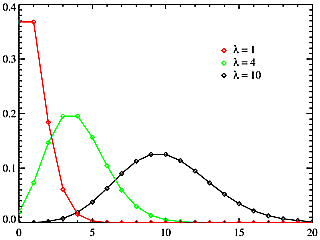## poisson

poisson — Poisson distribution random number generator (positive values only).

## Description

Poisson distribution random number generator (positive values only). This is an x-class noise generator.

## Syntax

`ares poisson klambda`
`ires poisson klambda`
`kres poisson klambda`

## Performance

ares, kres, ires - number of events occuring (always an integer).

klambda - the expected number of occurrences that occur during the rate interval.

In probability theory and statistics, the Poisson distribution is a discrete probability distribution. It expresses the probability of a number of events occurring in a fixed period of time if these events occur with a known average rate, and are independent of the time since the last event.

The Poisson distribution describing the probability that there are exactly k occurrences (k being a non-negative integer, k = 0, 1, 2, ...) is:where:

• λ is a positive real number, equal to the expected number of occurrences that occur during the given interval. For instance, if the events occur on average every 4 minutes, and you are interested in the number of events occurring in a 10 minute interval, you would use as model a Poisson distribution with λ = 10/4 = 2.5. This parameter is called klambda on the poisson opcodes.
• k refers to the number of i- , k- or a- periods elapsed.

The Poisson distribution arises in connection with Poisson processes. It applies to various phenomena of discrete nature (that is, those that may happen 0, 1, 2, 3, ... times during a given period of time or in a given area) whenever the probability of the phenomenon happening is constant in time or space. Examples of events that can be modelled as Poisson distributions include:

• The number of cars that pass through a certain point on a road (sufficiently distant from traffic lights) during a given period of time.
• The number of spelling mistakes one makes while typing a single page.
• The number of phone calls at a call center per minute.
• The number of times a web server is accessed per minute.
• The number of roadkill (animals killed) found per unit length of road.
• The number of mutations in a given stretch of DNA after a certain amount of radiation.
• The number of unstable nuclei that decayed within a given period of time in a piece of radioactive substance. The radioactivity of the substance will weaken with time, so the total time interval used in the model should be significantly less than the mean lifetime of the substance.
• The number of pine trees per unit area of mixed forest.
• The number of stars in a given volume of space.
• The distribution of visual receptor cells in the retina of the human eye.
• The number of viruses that can infect a cell in cell culture.A diagram showing the Poisson distribution.

For more detailed explanation of these distributions, see:

1. C. Dodge - T.A. Jerse 1985. Computer music. Schirmer books. pp.265 - 286

2. D. Lorrain. A panoply of stochastic cannons. In C. Roads, ed. 1989. Music machine . Cambridge, Massachusetts: MIT press, pp. 351 - 379.

## Examples

Here is an example of the poisson opcode. It uses the file poisson.csd. It is written for *NIX systems, and will generate errors on Windows.

Example 769. Example of the poisson opcode.

See the sections Real-time Audio and Command Line Flags for more information on using command line flags.

```<CsoundSynthesizer>
<CsOptions>
; Select audio/midi flags here according to platform
; Audio out   Audio in
; For Non-realtime ouput leave only the line below:
; -o poisson.wav -W ;;; for file output any platform
</CsOptions>
<CsInstruments>

; Initialize the global variables.
sr = 44100
ksmps = 441  ;ksmps set deliberately high to have few k-periods per second
nchnls = 1

; Instrument #1.
instr 1
; Generates a random number in a poisson distribution.
; klambda = 1

i1 poisson 1

print i1
endin

instr 2

kres poisson p4
printk (ksmps/sr),kres ;prints every k-period
endin

</CsInstruments>
<CsScore>

; Play Instrument #1 for one second.
i 1 0 1
i 2 1 0.2 0.5
i 2 2 0.2 4   ;average 4 events per k-period
i 2 3 0.2 20  ;average 20 events per k-period
e

</CsScore>
</CsoundSynthesizer>
```